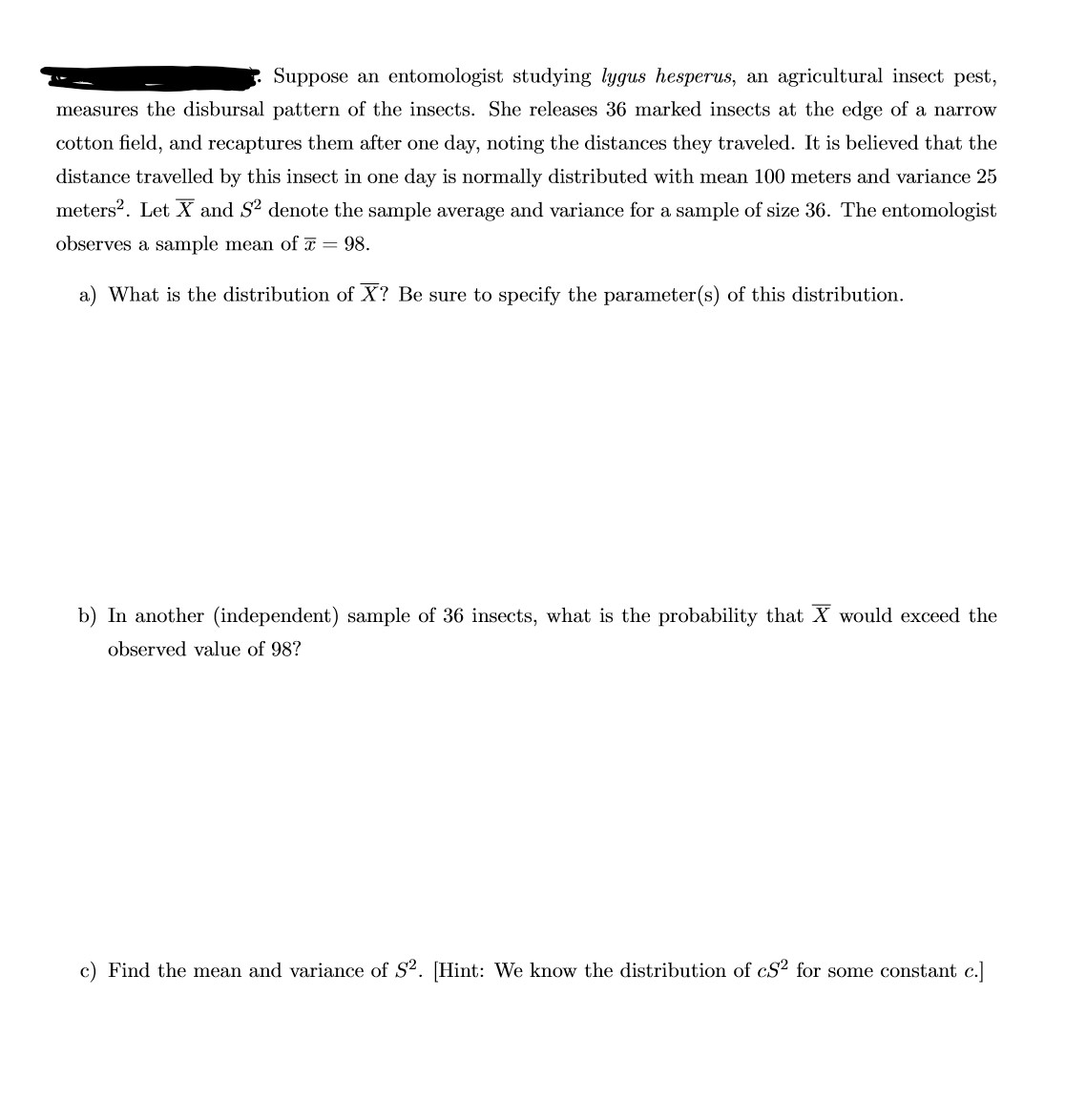# Suppose an entomologist studying lygus hesperus, an agricultural insect pest,measures the disbursal pattern of the insects. She releases 36 marked insects at the edge of a narrowcotton field, and recaptures them after one day, noting the distances they traveled. It is believed that thedistance travelled by this insect in one day is normally distributed with mean 100 meters and variance 25meters?. Let X and S2 denote the sample average and variance for a sample of size 36. The entomologistobserves a sample mean of = 98.a) What is the distribution of X? Be sure to specify the parameter(s) of this distribution.b) In another (independent) sample of 36 insects, what is the probability that X would exceed theobserved value of 98?c) Find the mean and variance of S2. [Hint: We know the distribution of cS2 for some constant c.]

Question
22 views

Part A of this Question,

In general what are the steps to finding the distribution of the Xbar?help_outlineImage TranscriptioncloseSuppose an entomologist studying lygus hesperus, an agricultural insect pest, measures the disbursal pattern of the insects. She releases 36 marked insects at the edge of a narrow cotton field, and recaptures them after one day, noting the distances they traveled. It is believed that the distance travelled by this insect in one day is normally distributed with mean 100 meters and variance 25 meters?. Let X and S2 denote the sample average and variance for a sample of size 36. The entomologist observes a sample mean of = 98. a) What is the distribution of X? Be sure to specify the parameter(s) of this distribution. b) In another (independent) sample of 36 insects, what is the probability that X would exceed the observed value of 98? c) Find the mean and variance of S2. [Hint: We know the distribution of cS2 for some constant c.] fullscreen
check_circle

star
star
star
star
star
1 Rating
Step 1

Points on sampling distribution of the sample mean:

• If the true population distribution of a random variable, say, x, is normal with parameters, mean μ and standard deviation σ, then, whatever be the size (n) of the sample taken from the population, the distribution of the sample mean is also normal, with parameters, mean μ and standard deviation σ/√n.
• Even if the true population distribution of a random variable, say, x, is not normal and has population mean μ, standard deviation σ, then, for a large size (n ≥ 30) of a sample taken from the popul...

### Want to see the full answer?

See Solution

#### Want to see this answer and more?

Solutions are written by subject experts who are available 24/7. Questions are typically answered within 1 hour.*

See Solution
*Response times may vary by subject and question.
Tagged in

### Other